# TS Grewal Solution Class 12 Chapter 3 Tools of Financial Statement Analysis 2020 2021

Read TS Grewal Solution Class 12 Chapter 3 Tools of Financial Statement Analysis 2020 2021 below, students should study TS Grewal class 12 Accountancy available on Studiestoday.com with solved questions and answers. These chapter wise answers for class 12 Accountancy have been prepared by teacher of Grade 12. These TS Grewal class 12 Solutions have been designed as per the latest NCERT syllabus for class 12 and if practiced thoroughly can help you to score good marks in standard 12 Accountancy class tests and examinations

Solutions for T.S. Grewal's Double Entry Book Keeping: Accounting for Not for Profit Organizations and Partnership Firms (Vol.3)
Textbook for CBSE Class 12
TS Grewal Solutions Class 12 Accountancy
Chapter 3
Tools of Financial Statement Analysis Comparative Statements and Common Size Statements

Question 1. From the following Balance Sheet, prepare Comparative Balance Sheet of Sun Ltd.: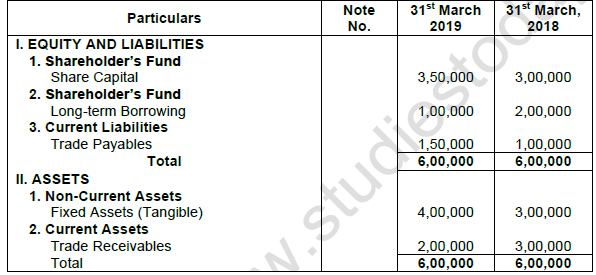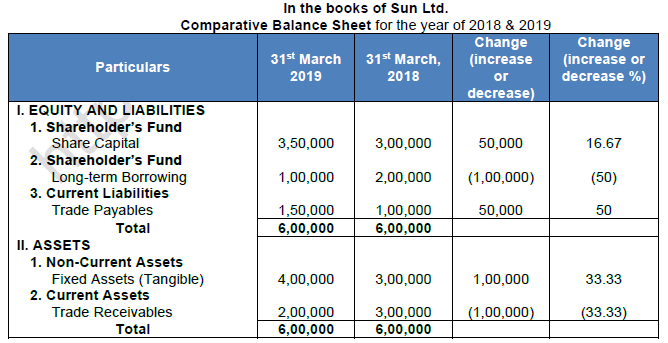Question 2. Following is the Balance Sheet, of Radha Ltd. as at 31st March, 2019: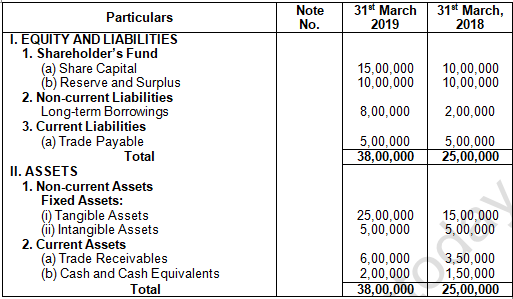You are required to prepare Comparative Balance Sheet on the basis of the information given in the above Balance Sheet.Question 3. Balance Sheet of Blue Bell Ltd. as at 31st March, 2019 is given below: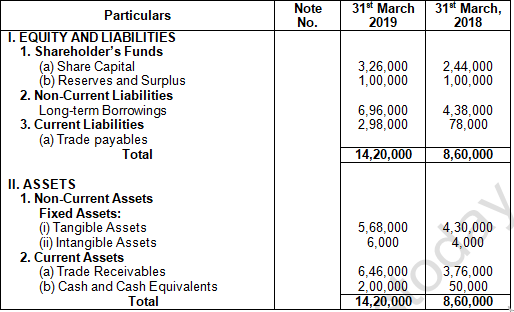You are required to prepare Comparative Balance Sheet on the basis of the information given in the above Balance Sheet.Question 4. Following is the summarised Balance Sheet of Wye Ltd. As at 31st March,2019: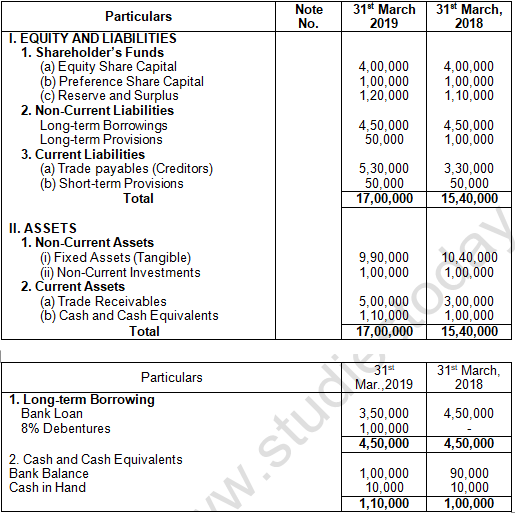You are required to comment upon the changes in absolute figures from one period to another.Question 5. From the Following Balance Sheet of Royal Industries Ltd. as at 31st March, 2019, prepare Comparative Balance Sheet: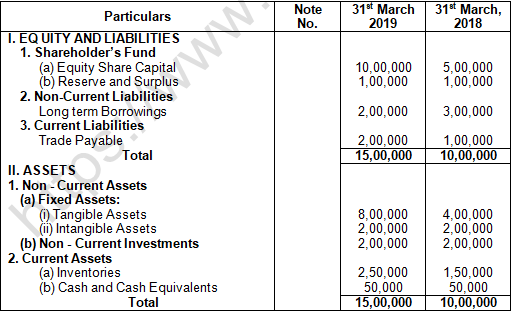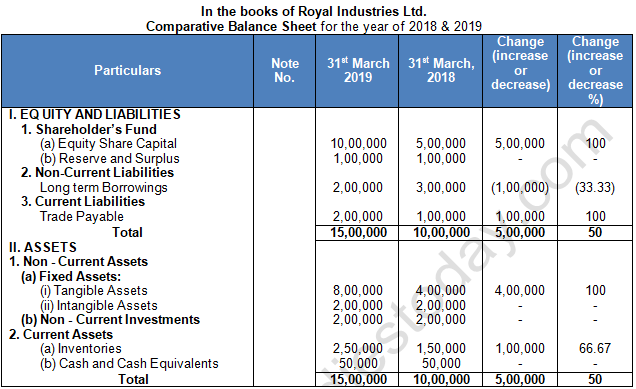Question 6. From the Following Balance Sheet of Depth Ltd. as at 31st March, 2019, prepare Comparative Balance Sheet: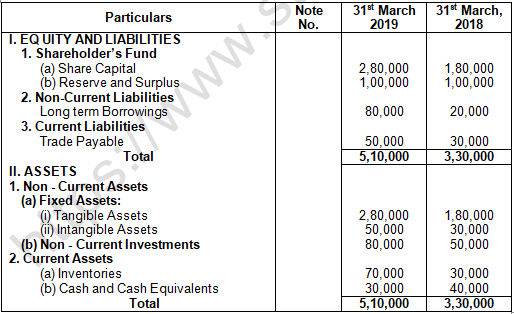Question 7. From the Following Balance Sheet of H.P. Ltd. as at 31st March, 2019, prepare Comparative Balance Sheet: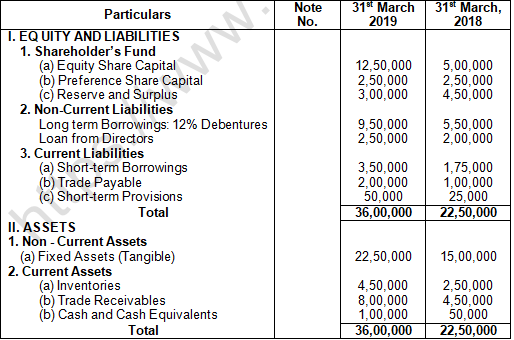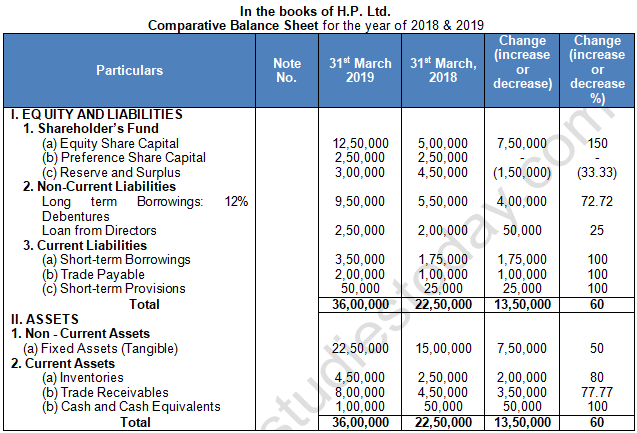Question 8. Following information is extracted from the Statement of Profit and Loss of Gold Coin Ltd. for the year ended 31st March, 2015Question 9. Prepare Comparative Statement of Profit and Loss from the following Statement of Profit and Loss: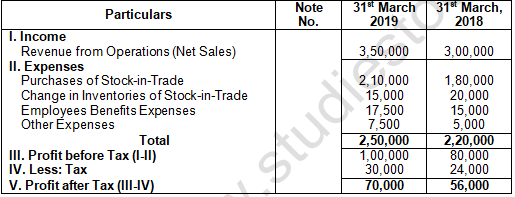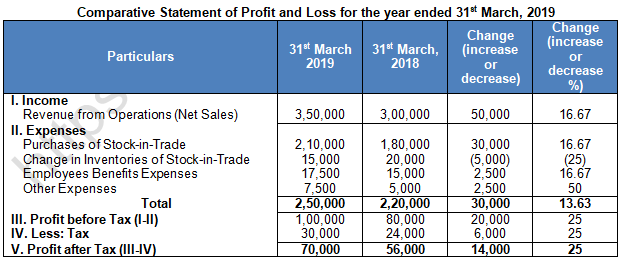Question 10. Prepare Comparative Statement of Profit and Loss from the following Statement of Profit and Loss:Question 11. From the following Information, prepare Comparative Statement of Profit and Loss: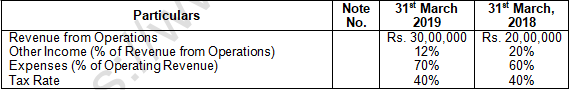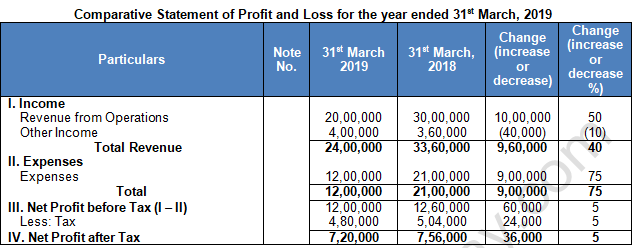Working Note:-

1. Calculation of Other Incomes for 2019 = Rs. 20,00,000 × 20% = Rs. 4,00,000

2. Calculation of Other Incomes for 2018 = Rs. 30,00,000 × 12% = Rs. 3,60,000

3. Calculation of Expenses for 2019 = Rs. 20,00,000 × 60% = Rs. 12,00,000

4. Calculation of Expenses for 2018 = Rs. 30,00,000 × 70% = Rs. 21,00,000

Question 12. From the following information, prepare comparative statement of profit and loss showing increase, decrease and percentage: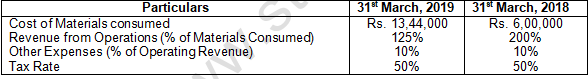Working Note:-

1. Calculation of Revenue from Operation for 2019 = 13,44,000 × 125/100 = 16,80,000
2. Calculation of Revenue from Operation for 2018 = 6,00,000 × 200/100 = 12,00,000
3. Calculation of Other Expenses for 2019 = 16,80,000 × 10/100 = 1,68,000
4. Calculation of Other Expenses for 2018 = 12,00,000 × 10/100 = 1,20,000
5. Calculation of Tax for 2019 = 1,68,000 × 50% = 84,000
6. Calculation of Tax for 2018 = 4,80,000 × 50% = 2,40,000

Question 13. From the following Statement of Profit and Loss, prepare comparative statement of profit and loss: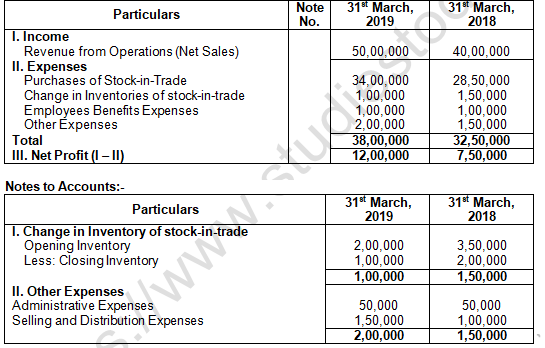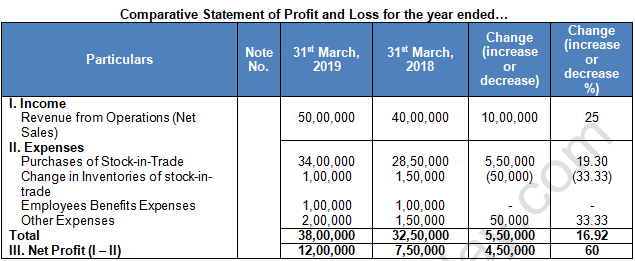Question 14. Prepare Comparative Statement of Profit and Loss from the following information: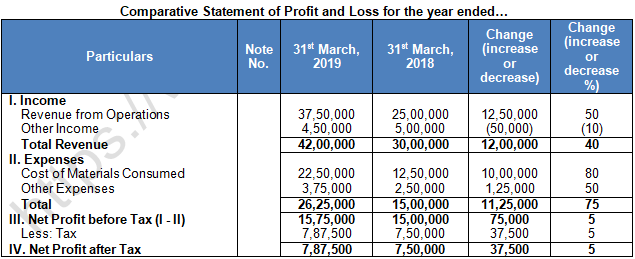Question 15. From the following Statement of Profit and Loss of Antriksh Ltd. for the year ended 31st March, 2012 and 2013, Prepare Comparative Statement of Profit and Loss:Rate of Income Tax was 40%.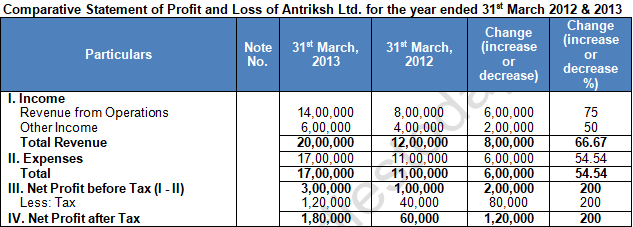Question 16. From the following Statement of Profit and Loss, prepare Comparative Statement of Profit and Loss: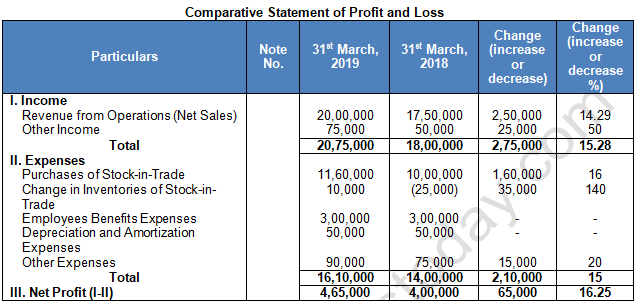Question 17. From the following Statement of Profit and Loss of Star Ltd., for the year ended 31st March, 2015 and 2016, prepare a Common size statement: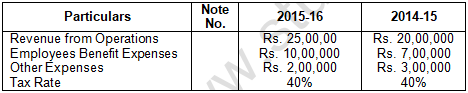Question 18. Prepare Common-size Statement of Profit and Loss from the following statement of Profit and Loss: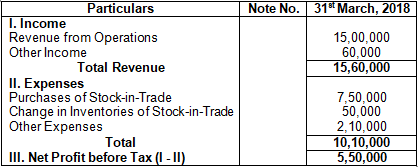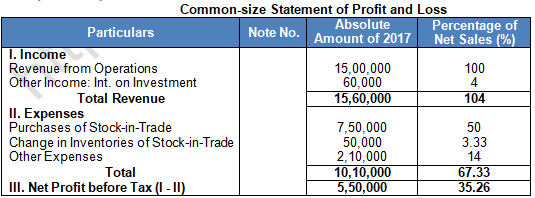Question 19. Prepare Common-size Statement of Profit and Loss from the following statement of Profit and Loss: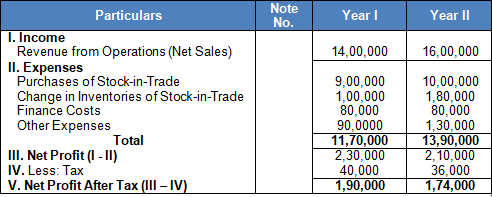Common-size income statement for the years ended I and II.Question 20. Prepare Common-size Balance Sheet and comment on the financial position of Sun Ltd. and Star Ltd. The Balance Sheet of Sun Ltd. and Star Ltd. as at 31st March, 2019 are:## Tags:

Click for more Accountancy Study Material

## Latest NCERT & CBSE News

Read the latest news and announcements from NCERT and CBSE below. Important updates relating to your studies which will help you to keep yourself updated with latest happenings in school level education. Keep yourself updated with all latest news and also read articles from teachers which will help you to improve your studies, increase motivation level and promote faster learning